# Getting Started with imagerExtara

#### 2019-01-25

imagerExtra provides advanced functions for image processing based on the R package imager.

The functions in imagerExtra are classified into 4 groups by their functions.

• Contrast Enhancement
• Segmentation
• Denoising
• Others

See below to know what functions imagerExtra provides.

## Preparation

Most of the functions in imagerExtra are for grayscale image.

See the vignette Treating Color Image with imagerExtra if you want to treat color image with imagerExtra.

Let’s prepare grayscale images.

library(imagerExtra)
g <- grayscale(boats)
gd <- grayscale(dogs)
layout(matrix(1:2,1,2))
plot(g, main = "boats")
plot(gd, main = "dogs")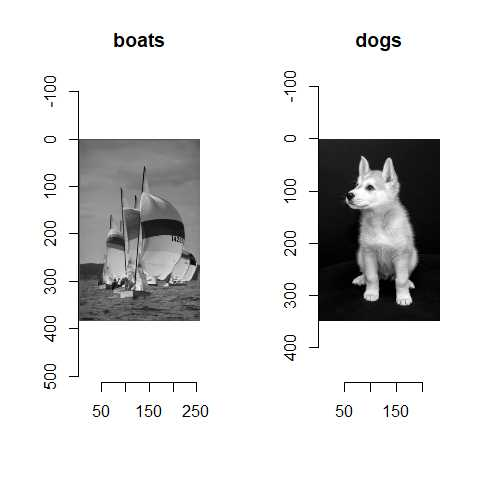## Contrast Enhancement

The functions for contrast enhancement are

• EqualizePiecewise
• BalanceSimplest
• SPE
• EqualizeDP
• EqualizeADP

### EqualizePiecewise (Piecewise Affine Histogram Equalization)

EqualizePiecewise has three parameters: N, smin, and smax.

However, we should not change smin.

See Jose-Luis Lisani, et al., IPOL, 2 (2012), pp. 243-265. for detail.

The parameter N controls how the input gray level will be mapped in the output image.

We don’t have a priori choice for N. You will tune N mainly.

The parameter smax controls the upper limit of contrast stretching.

If you want to prevent excessive enhancement of contrast, you should make smax low.

layout(matrix(1:4, 2, 2))
plot(g, main = "Original")
EqualizePiecewise(g, 2) %>% plot(main = "N = 2")
EqualizePiecewise(g, 10) %>% plot(main = "N = 10")
EqualizePiecewise(g, 1000) %>% plot(main = "N = 1000")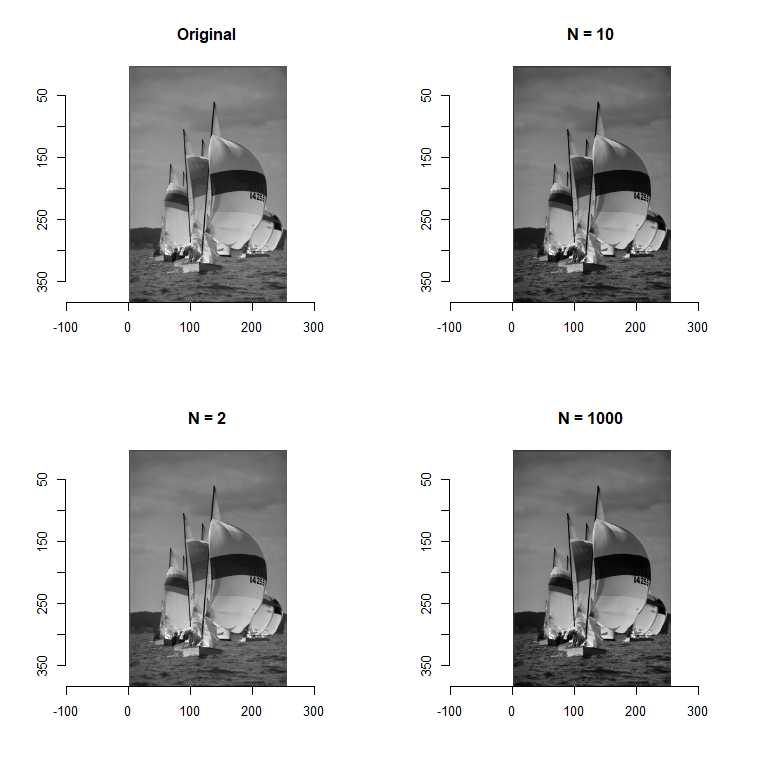### BalanceSimplest (Simplest Color Balance)

BalanceSimplest saturates a percentage sleft % of the pixels on the left side of the histogram,

and a percentage sright % of the pixels on the right side of the histogram.

See Nicolas Limare, et al., IPOL, 1 (2011), pp. 297-315. for detail.

layout(matrix(1:2, 1, 2))
plot(g, main = "Original")
BalanceSimplest(g, 1, 1) %>% plot(main = "sleft = 1, sright = 1")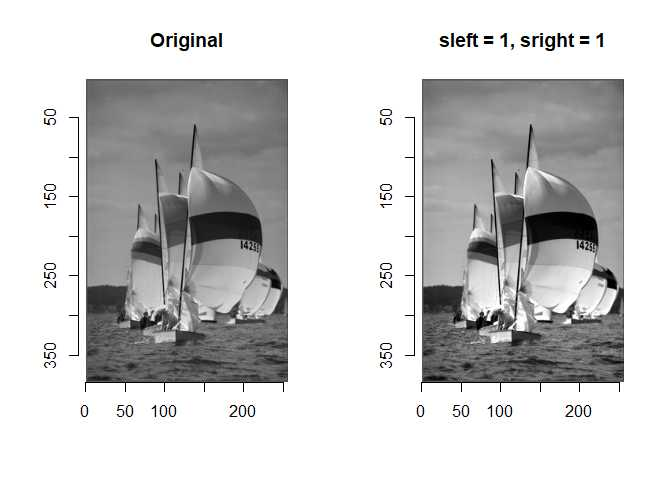### SPE (Screened Poisson Equation)

The distinction of SPE is that SPE corrects the inhomogeneous background of image.

See Jean-Michel Morel, et al., IPOL, 4 (2014), pp. 16-29. for detail.

The parameter lamda controls how strong corrects inhomogeneous background of image.

SPE corrects inhomogeneous background strongly if lamda is large.

layout(matrix(1:2, 1, 2))
plot(papers, main = "Original")
SPE(papers, 0.1) %>% plot(main = "SPE (lamda = 0.1)")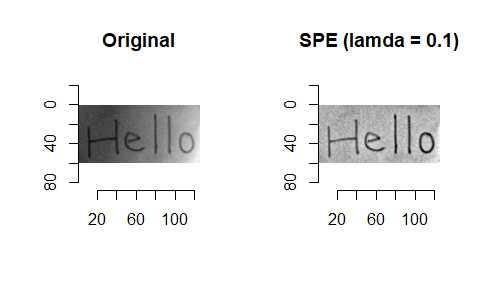### EqualizeDP (Double Plateaus Histogram Equalization)

Double plateaus histogram equalization (DPHE) enhances contrast of image while preventing over-enhancement of background noise and protecting details of objects in images.

DPHE modifies original histogram as shown below, and then apply histogram equalization. $H_{m}(g) = \begin{cases} T_{UP} & h(g) >= T_{UP} \\ h(g) & T_{DOWN} < h(g) < T_{UP} \\ T_{DOWN} & h(g) <= T_{DOWN} \end{cases}$ where $$g$$ is the gray level, $$h(g)$$ is the original histogram, and $$H_{m}(g)$$ is the modified histogram.

You will tune the two key parameters, $$T_{DOWN}$$ and $$T_{UP}$$.

layout(matrix(1:2, 1, 2))
plot(gd, main = "Original")
EqualizeDP(gd, 25, 110) %>% plot(main = "DPHE")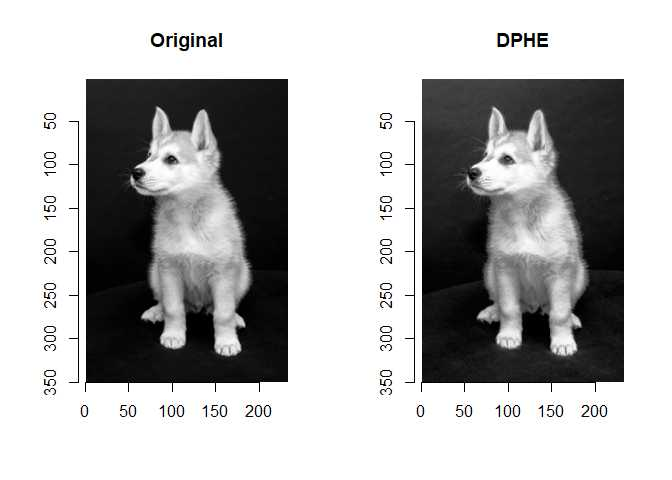### EqualizeADP (Adaptive Double Plateaus Histogram Equalization)

Adaptive double plateaus histogram equalization (ADPHE) computes $$T_{DOWN}$$ and $$T_{UP}$$ automatically, and then apply DPHE.

layout(matrix(1:2, 1, 2))
plot(gd, main = "Original")
EqualizeADP(gd) %>% plot(main = "ADPHE")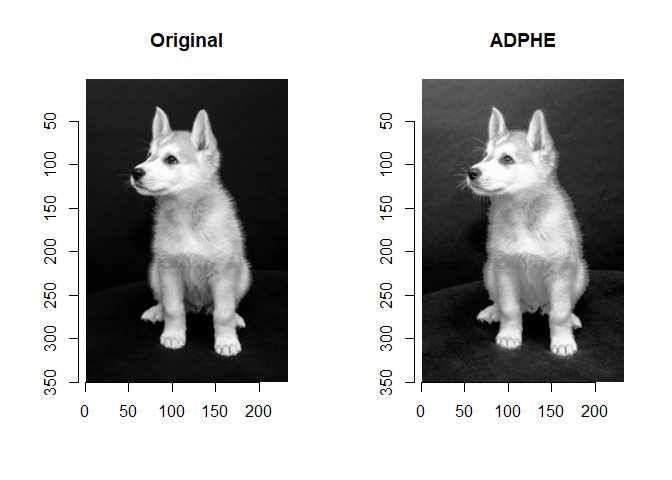## Denoising

The function for image denoising is

• DenoiseDCT

### DenoiseDCT (DCT denoising)

DCT denoising is a simple and effective denoising algorithm using local DCT thresholding.

The parameter sdn determines how strong denoise a image.

Noise is strongly denoised if sdn is large.

The parameter flag_dct16x16 determiens window size of local patches.

DenoiseDCT uses 8x8 windows or 16x16 window.

Larger window size does not bring significant improvement when noise level is low.

Larger window size outperforms significantly smaller window size when noise level is low.

noisy <- g + imnoise(dim = dim(g), sd = 0.1)
layout(matrix(c(1,3,2,4), 2, 2))
plot(g, main = "Original")
plot(noisy, main = "Noisy Boats")
DenoiseDCT(noisy, 0.1) %>% plot(., main = "Denoised (8x8 window)")
DenoiseDCT(noisy, 0.1, flag_dct16x16 = TRUE) %>% plot(., main = "Denoised (16x16 window)")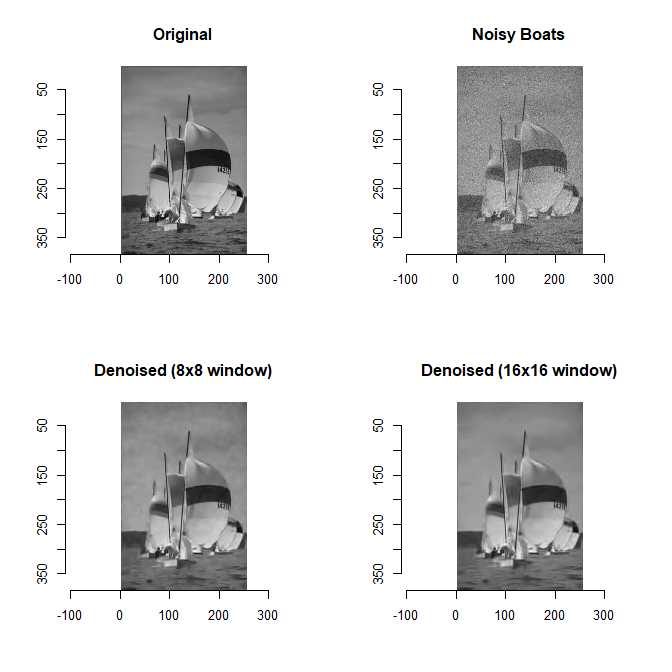## Segmentation

The functions for image segmentation are

• ThresholdTriclass
• ThresholdAdaptive
• ThresholdFuzzy
• ThresholdML
• SegmentCV

### ThresholdTriclass (Iterative Triclass Thresholding)

Iterative triclass thresholding is an iterative thresholding technique.

We need to set a rule to stop iteration.

We have two options.

• set preset threshold (stopval)
• set repeat number (repeatnum)
gdogs <- grayscale(dogs)
layout(matrix(1:4, 2, 2, byrow = TRUE))
plot(gdogs, main = "Original", axes=F)
ThresholdTriclass(gdogs, stopval = 0.001) %>% plot(main = "stopval = 0.001")
ThresholdTriclass(gdogs, repeatnum = 1) %>% plot(main = "repeatnum = 1")
ThresholdTriclass(gdogs, repeatnum = 3) %>% plot(main = "repeatnum = 3")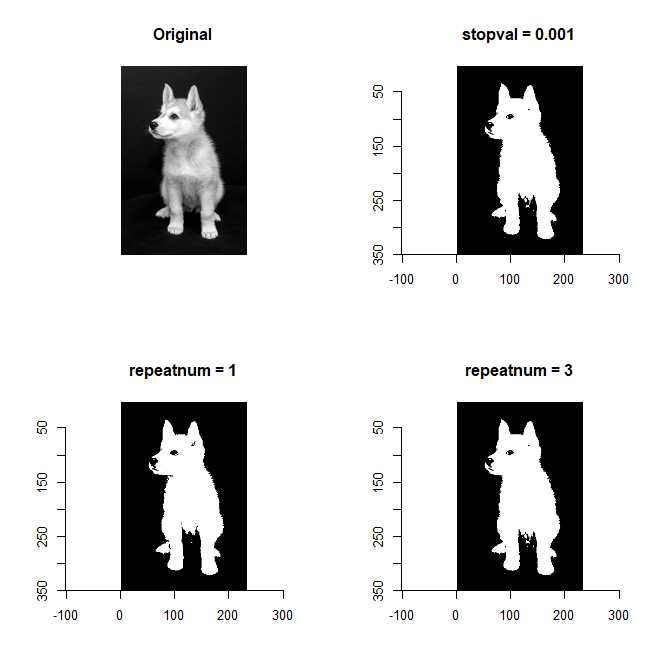### ThresholdAdaptive (Local Adaptive Thresholding)

Local adaptive thresholding can extract objects from inhomogeneous background.

You will tune the two paramters k and windowsize.

Note that the parameter range determines max standard deviation.

you should set range as [0,1] if you treat a image whose pixel values are in [0,1].

layout(matrix(1:2,1,2))
plot(papers, main = "Original")
hello <- ThresholdAdaptive(papers, 0.1, windowsize = 17, range = c(0,1))
plot(hello, main = "Binarizesd")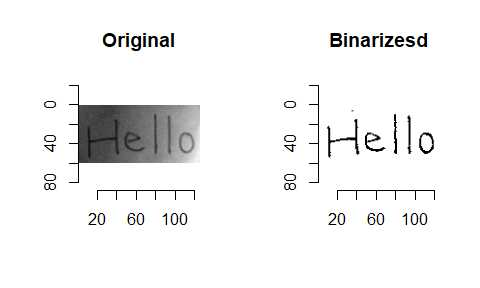### ThresholdFuzzy (Fuzzy Thresholding)

Fuzzy thresholding is an automatic thresholding based on fuzzy set theory.

layout(matrix(1:2,1,2))
plot(g, main = "Original")
ThresholdFuzzy(g) %>% plot(main = "Fuzzy Thresholding")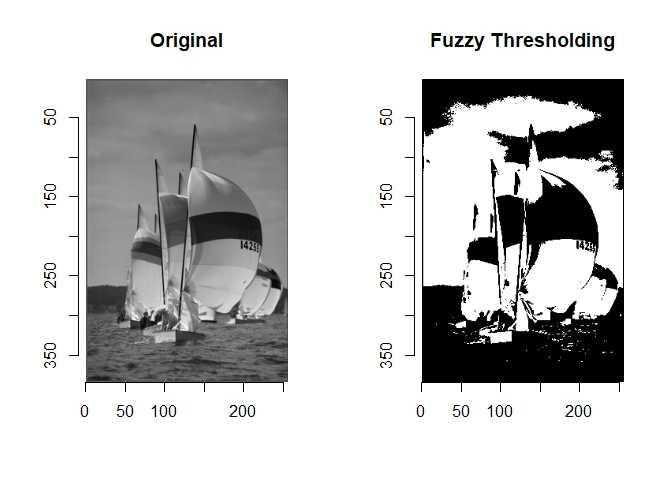### ThresholdML (Multilevel Thresholding)

Multilevel thresholding segments an image into several gray levels.

You can specify level of thresholds or values of thresholds.

The values of thresholds are computed automatically if you specify the level of thresholds.

layout(matrix(1:2,1,2))
ThresholdML(g, k = 3) %>% plot(main = "Level of Thresholds: 3")
ThresholdML(g, thr = c(0.2, 0.4, 0.6)) %>% plot(main = "Thresholds: 0.2, 0.4, and 0.6")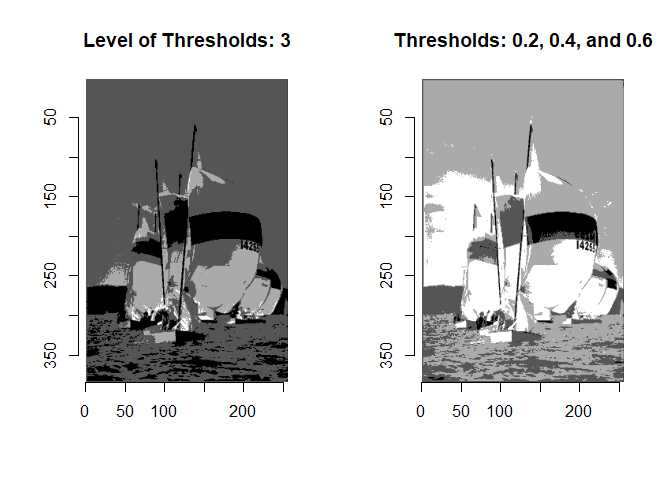### SegmentCV (Chan-Vese Segmentation)

Chan-Vese segmentation (CVS) is an iterative region-based segmentation algorithm.

CVS can extract objects whose pixel values aren’t homogeneous.

This is the distinction of CVS.

SegmentCV has many arguments and most of the arguments are key paramters.

It’s too many to explain briefly.

layout(matrix(1:2, 1, 2))
plot(gd, main = "Original")
SegmentCV(gd, lambda2 = 15) %>% plot(main = "Chan-Vese")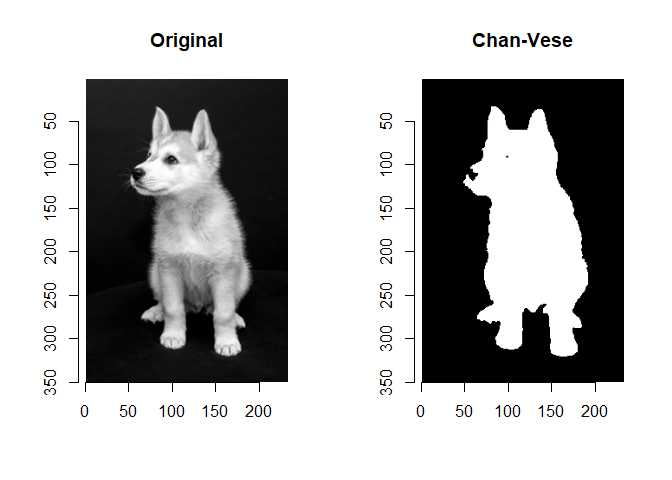## Others

The functions classified as others are

• Grayscale
• GetHue
• RestoreHue
• OCR
• OCR_data

### Grayscale, GetHue, and RestoreHue

These functions are for treating color image with imagerExtra.

See the vignette Treating Color Image with imagerExtra for detail.

### OCR and OCR_data

These functions are wrappers for ocr function and ocr_data function of the R package tesseract.

See the vignette Optical Character Recognition with imagerExtra for detail.